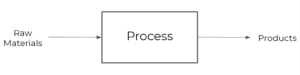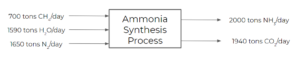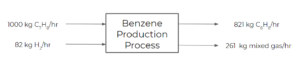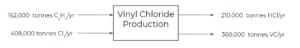# 4 Input-Output Diagrams

Learning Objectives

By the end of this section, you should be able to:

Create input-output diagrams

## Input-Output Diagrams

These diagrams are considered to be the simplest of process flow sheets. In input-output diagrams, the entire process is represented by 1 block only. They usually only reference the main process streams in and out of a process.Example: Ammonia Production Input-output Diagram

Consider an ammonia production process where 3 main streams of raw materials enter the process:

• 700 tons/day of methane
• 1590 tons/day of water
• 1650 tons/day of nitrogen

The process produces 2 main product streams:

• 2000 tons/day of ammonia
• 1940 tons/day of carbon dioxide

We can put together the following input-output diagram for this process:Exercise: Benzene Production Input-output Diagram

Consider a process where toluene is fed into a process at 1000 kg/hr and hydrogen is fed at 82 kg/hr. The following reaction takes place in the process:

$C_{7}H_{8} + H_{2} ➡ C_{6}H_{6} + CH_{4}$

The reaction achieves 75% conversion and the products of the process are separated into 2 streams. The first product stream is benzene, exiting at 821 kg/hr. The second product stream is a mixed gas stream (including the unreacted reactants and by-products) exiting at 261 kg/hr. Draw an input-output diagram for this process.

### SolutionExercise: Production of Vinyl Chloride

Our client wants to produce 360,000 tonnes/yr (800 million lbs/yr) of vinyl chloride monomer. We decide to use the following reaction pathway, which contains two reaction steps as follows:

$C_{2}H_{4} + Cl_{2} → C_{2}H_{4}Cl_{2} (1)$

$C_{2}H_{4}Cl_{2} → C_{2}H_{3}Cl + HCl (2)$

We gather the following information on the process:

Name Formula Molecular Weight (g/mol) Price (USD/tonne)
Ethene $C_{2}H_{4}$ 28.05 733
Chlorine $Cl_{2}$ 70.91 240
Dichloroethane $C_{2}H_{4}Cl_{2}$ 98.96 300
Hydrogen Chloride $HCl$ 36.46 140
Vinyl Chloride $C_{2}H_{3}Cl$ 62.50 750

Assume as well that our production costs are $200\frac{USD}{tonne}$ of VC produced, and assume our VC production plant costs $50,000,000 USD$ to build. Note these values usually depend on the size of the plant, location, and other factors.

Create an input-output diagram of the process.

### Solution

We know that one of the product streams will be 360,000 tonnes/yr of vinyl chloride. Hydrogen chloride is produced in a 1:1 molar ratio with vinyl chloride:

$VC = 360,000 tonnes/yr*\frac{tonne-mol}{62.50 tonnes} = 5,760\frac{tonne-mol}{yr}$

$HCl = 5,760\frac{tonne-mol}{yr}*36.46\frac{tonnes}{tonne-mol}=210,000\frac{tonnes}{yr}$

Similarly for ethane and chlorine, we get:

$C_{2}H_{4} = 5,760\frac{tonne-mol}{yr}*28.05\frac{tonnes}{tonne-mol}=162,000\frac{tonnes}{yr}$

$Cl_{2} = 5,760\frac{tonne-mol}{yr}*70.91\frac{tonnes}{tonne-mol}=408,000\frac{tonnes}{yr}$

From this and the information in the table, we get the following input-output diagram: